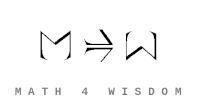文章 发现 ms@ms.lt +370 607 27 665 My work is in the Public Domain for all to share freely. 读物 书 影片 维基百科 Software Upload Transfer notes MathNotes, Simplex 数学 _ _ _ _ מאטעמאטיק Math research An overview of my research. Math notebook Questions that I am investigating. Andrius Kulikauskas: I wish to relate my philosophy and mathematics in fruitful ways. Math connections Express my philosophy's concepts in terms of mathematics and thus better define my concepts as well as understand which mathematical concepts are most central. Coincidences Note mathematical coincidences that may bear on my philosophical structures. Math big picture Understand the entirety of math. The purpose of math Understand what distinguishes math from other languages and disciplines. Math discovery Uncover the system by which things are figured out in mathematics. Map of math Show how math unfolds, how its various branches and concepts arise. Math Companion Organize concepts from The Princeton Companion to Mathematics. Basic math Formulate and appreciate the most basic mathematical principles. Implicit math Understand the metaphysical roots of math as a cognitive language that we experience. Math dimensions Distinguish and investigate the many dimensions of math, such as beauty, history, education, insight, learning, humanity. Study math Learn what is most relevant. MathExercises Document the exercises that help me learn and investigate. Collaboration. Collaborate with others on new ways of thinking about math.
Šis puslapis paskutinį kartą keistas April 13, 2020, at 11:39 AM